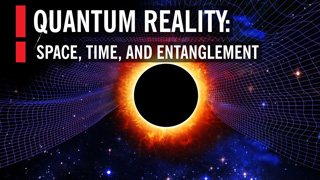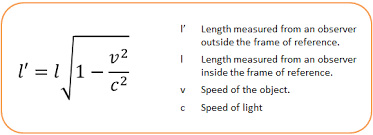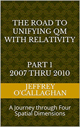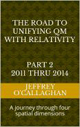# The Reality of the Quantum Universe

Quantum mechanics defines the position of all particles in the universe only in terms of the probabilistic values associated with Schrodinger’s wave equation.  In other words, it tells us that because they are defined in terms probabilities they are randomly distributed throughout the entire universe before being observed.

However, Einstein disagreed.  He felt one could explain those probabilities in terms of the observable properties of the physical universe.  In other words, he felt because it would be more natural or probable to observe a particle or objects to be located at, or, at the very least, near where it’s found a moment later instead of assuming they are spread out over the entire universe as Quantum mechanics does.Â  If that is the case, a deeper understanding of physics should provide that information which will contradict that aspect of quantum mechanics.ÂThe most logical way to determine if this is possible would be to integrate the observable properties of particles with those of our physical universe to see if one can explain them.

This would be true even though we cannot observe an individual quantum particle because there are properties of it that we can observe.

For example, as was mentioned earlier quantum mechanics defines the structure of the universe in terms of the abstract probabilities associated with Schrodinger’s wave equation which only become physical or non-abstract when observed.  This is why it assumes that our universe has a dual existence; the physical one associated with particles and the non-physical or probabilistic one associated with Schrodinger’s wave equation.  Additionally, it assumes that when an observation is made that equation and the probabilities associated with its “collapse” to create physical universe as we know it at the time of the observation.

But the physicality of its wave component was confirmed in 1927 by Davisson and Germer by the observation that electrons and later all particles can be diffracted by crystals. This provides observational verification of physicality of the wave/particle duality of Quantum Mechanics associates with both particles and the universe because the only way to explain the diffraction pattern produce is to assume that that they are made up of electromagnetic waves.

However, it showed the successes of Schrodinger’s wave equation may not be based purely on the mathematics of probabilities but on the physical properties of the energy wave observed by Davisson and Germer.

Yet, before we can understand why, we must first view the universe in terms of the spatial properties of position to understand how the physical properties of that wave interacts with it to define its position when observed. In others words to show why “it would be more natural or probable to observe a particle to be located at, or, at the very least, near where it’s found a moment later one must define the universe in terms of its spatial instead of its time properties, as Einstein had done in his Special and General theories of relativity.

Einstein gave us the ability to do this when he defined the geometric properties of space-time in terms of the constant velocity of light because that provided a method of converting a unit of time in a space-time environment of unit of space in four *spatial* dimensions. Additionally, because the velocity of light is constant, he also defined a one to one quantitative and qualitative correspondence between his space-time universe and one made up of four *spatial* dimensions.

Doing so allows one to understand how the randomness of a particles position, as defined by quantum mechanics is not dependent on the abstract properties of Schrodinger’s wave equation but on the physical properties of the universe as define by Einstein.

For example, the article, “Why is energy/mass quantized?” Oct. 4, 2007 showed that one can use the spatial equivalent of Einstein’s theories, defined above to explain the quantum mechanical properties of an electromagnetic wave by extrapolating the rules of classical resonance in a three-dimensional environment to the energy wave discovered by Davisson and Germer moving on the “surface” of a three-dimensional space manifold with respect to a fourth *spatial* dimension.

Briefly it showed the four conditions required for resonance to occur in a classical environment, an object, or substance with a natural frequency, a forcing function at the same frequency as the natural frequency, the lack of a damping frequency and the ability for the substance to oscillate spatial would occur in an energy wave moving in four *spatial* dimensions.

The existence of four *spatial* dimensions would give an energy of the wave, mentioned earlier Davisson and Germer discovered the ability to oscillate spatially on a “surface” between a third and fourth *spatial* dimension thereby fulfilling one of the requirements for classical resonance to occur.

These oscillations would be caused by an event such as the decay of a subatomic particle or the shifting of an electron in an atomic orbital.Â This would force the “surface” of a three-dimensional space manifold with respect to a fourth *spatial* dimension to oscillate with the frequency associated with the energy of that event.
However, the oscillations caused by such an event would serve as forcing function allowing a resonant system or “structure” to be established on a surface of a three-dimensional space manifold.
Yet the classical laws of three-dimensional space tell us the energy of resonant systems can only take on the discontinuous or discreet energies associated with their fundamental or harmonic of their fundamental frequency.

However, these are the similar to the quantum mechanical properties of energy/mass in that they can only take on the discontinuous or discreet energies associated with the formula E=hv where “E” equals the energy of a particle “h” equal Planck’s constant “v” equals the frequency of its wave component.
Yet it also allowed us to define the physical boundaries of a quantum system in terms of the geometric properties of four *spatial* dimensions.

In physics, a point on the two-dimensional surface of paper is confined to that surface. However, that surface can oscillate up or down with respect to three-dimensional space.
Similarly, an object occupying a volume of three-dimensional space would be confined to it however, it could, similar to the surface of the paper oscillate “up” or “down” with respect to a fourth *spatial* dimension.

The confinement of the “upward” and “downward” oscillations of a three-dimension volume with respect to a fourth *spatial* dimension is what defines the spatial boundaries associated with a particle in the article “Why is energy/mass quantized?”.
In other words, the energy wave in four *spatial* dimensions associated with the dual particle/wave properties of existence define by quantum mechanics will maintain its wave properties unless it is confined to three by an observation, then it will be observed as particle

This suggests that it is not the abstract properties of Schrodinger’s wave equation that collapses when an observation is made but instead it is the collapse of the wave energy observed by Davisson and Germer when confined to three-dimensional space by an observation.e

The physics of wave mechanics also tells us that due to their continuous properties the energy waves the article “Why is energy/mass quantized?” Oct. 4, 2007 associated with a quantum system would be distributed throughout the entire “surface” a three-dimensional space manifold with respect to a fourth *spatial* dimension.

For example, the energy of a vibrating or oscillating ball on a rubber diaphragm would be disturbed over its entire surface while the magnitude of those vibrations would decrease as one move away from the focal point of the oscillations.

Similarly, if the assumption that quantum properties of energy are a result of vibrations or oscillations in a “surface” of three-dimensional space is correct those oscillations would be distributed over the entire “surface” three-dimensional space while the magnitude of those vibrations would be greatest at the focal point of the oscillations and decreases as one moves away from it.(Some may question the fact that the energy wave associated with particle would be distributed over the entire universe.  However, the relativistic properties of space-time and four *spatial* dimensions tell us that distance perceived by objects or particles in relative motion is dependent on their velocity which become zero at the speed of light.Â  Therefore, from the perspective of an electromagnetic wave moving at the speed of light, the distance between all points in the universe along its velocity vector is zero.Â  In other words, its energy is distributed or simultaneous exists at every point in the universe along its velocity vector.Â  There can be no other conclusion if one accepts the validity of Einstein’s theories.)

As mentioned earlier the article “Why is energy/mass quantized?â” shown a quantum particle is a result of a resonant structure formed by an energy wave on the “surface” of a three-dimensional space manifold with respect to a fourth *spatial* dimension.

Yet, the science of Wave Mechanics tells us resonance would most probably occur on the surface of the rubber sheet were the magnitude of the vibrations is greatest and would diminish as one move away from that point.

Similarly, a particle would most probably be observed were the magnitude of the vibrations in a “surface” of a three-dimensional space manifold is greatest and would diminish as one move away from that point.

Yet, observations of the physical world around us tell that point will most likely not be very different from where it was a moment ago.

However this also tell us because of the physicals properties of the wave/particle component of the universe they will have a definite position relative to each other.

As was mentioned earlier, the probabilistic interoperation of Schrodinger’s wave equation tells us that all the particles in the universe are randomly disturbed before being observed.

However, as was show above, one could argue it only defines the probability of finding the position were the collapse of the wave associated with each individual particle occurs.Â  In other words, contrary to currently accepted Quantum Mechanical interpretation, the individual particle/wave components of the universe are not randomly disturbed because, as was shown aboveÂ they would have a definite position relative to each other before it is observed.

Therefore, Einstein assumption, mentioned earlier that “it would be more natural or probable to observe a particle to be located at, or, at the very least, near where it’s found a moment earlier would be consistent with that probabilistic interpretation of Quantum Mechanics.Â Because, as the above discussion shows one cannot, in a universe governed by its wave/particle duality and Relativity know exactly were a particle will be when observed.

in other words, “The Reality of the Quantum Universe” can be understood in terms of is wave/particle duality and the space-time environment defined by Einstein.

It should be remembered Einstein’s genius allows us to choose whether to define the probabilities in Quantum Mechanics in either a space-time environment or one consisting of only four *spatial* dimension when he defined its geometry in terms of the constant velocity of light.

Latter Jeff

 The Road to Unifying QM with Relativity part 1 2007 thru 2010Ebook \$8.00Paper Back \$15.00 The Road to Unifying QM with Relativity part 2 2011 thru 2014Ebook \$8.00Paper Back \$16.00 The Road to Unifying QM with Relativity part 3 2015 thru 2020Ebook \$8.00Paper Back \$18.00